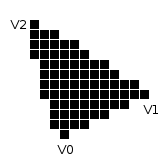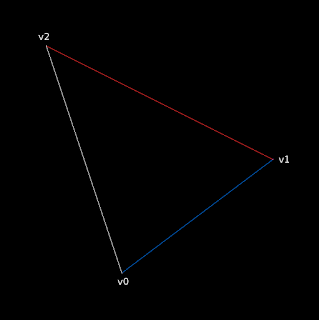## Saturday, August 12, 2017

### Triangle rasterization under the hood (the old-school approach)

I didn't find a single source which would gave me a complete and clear picture of how to rasterize a triangle in a simplest way.

So, here's my attempt to put everything in order.

Consider, that we know how to draw a line and nothing more. Here's the corresponding method declaration for it:

void drawLine(int x0, int y0, int x1, int y1, Color color);

We need to implement a method which will draw a triangle and fill it with a specified color. Here's the corresponding method declaration:

void drawTriangle(Vec2 v0, Vec2 v1, Vec2 v2, Color color);

Here's the visual representation of what this method should do:Initial verticesRasterized triangle

drawTriangle(v0, v1, v2, Color.BLACK)
============================>

Here's initial triangle we're going to fill in:

void drawTriangle(Vec2 v0, Vec2 v1, Vec2 v2, Color color)
{
drawLine(v0.x, v0.y, v1.x, v1.y, blue);
drawLine(v1.x, v1.y, v2.x, v2.y, red);
drawLine(v2.x, v2.y, v0.x, v0.y, white);
}

We need to sort all vertices by y coordinate. Thus, we will know the longest triangle side and two short sides. Let's do this:void drawTriangle(Vec2 v0, Vec2 v1, Vec2 v2, Color color)
{
if (v2.y < v0.y) swap(v2, v0);
if (v2.y < v1.y) swap(v2, v1);
if (v1.y < v0.y) swap(v1, v0);

drawLine(v0.x, v0.y, v1.x, v1.y, blue);
drawLine(v1.x, v1.y, v2.x, v2.y, red);
drawLine(v2.x, v2.y, v0.x, v0.y, white);
}

After applying this kind of sorting we will have vertices sorted as follows: v0.y <= v1.y <= v2.y as you can see in the picture to the right.

The next step is to draw horizontal lines between the longest edge and two short edges. So, we need to fill in two internal triangles separately. To be more clear, let's look at the image:

here, lines between (v0, v2) and (v0, v1) edges are in a blue color and form an internal blue triangle, and lines between (v0, v2) and (v1, v2) edges are in a red color and form an internal red triangle.

And now the most interesting part begins: how to find those horizontal lines (let's call them spans)? To answer this question we need to refresh our knowledge about linear interpolation. Wikipedia can help us to do this :)

Given the two red points, the blue line is the linear interpolant between the points, and the value y at x may be found by linear interpolation.

If the two known points are given by the coordinates (x0, y0) and (x1, y1), the linear interpolant is the straight line between these points.

Here's the main equation for linear interpolation:

(y - y0) / (x - x0) = (y1 - y0) / (x1 - x0)

Let's come back to our triangle and consider the internal blue triangle. A span in this triangle starts on the edge (v0, v2) and ends on the edge (v0, v1). We already have y coordinate for the span, where y is in the range [v0.y, v1.y]. It will be the same for both span's ends because the span is a horizontal line. If we iterate through the interval [v0.y, v1.y] we can find the needed x values for the span's ends. And that's it, we have all needed to draw the span.

We can use the aforementioned equation for linear interpolation to find those x values. Let's get equations for edges (v0, v2) and (v0, v1):

(v0, v2): (y - v0.y) / (v0.x - x0) = (v2.y - v0.y) / (v0.x - v2.x)
(v0, v1): (y - v0.y) / (x1 - v0.x) = (v1.y - v0.y) / (v1.x - v0.x)

Apply some simple math and get equations for x0 and x1:

x0 = v0.x - (y - v0.y) * ((v0.x - v2.x) / (v2.y - v0.y))
x1 = v0.x + (y - v0.y) * ((v1.x - v0.x) / (v1.y - v0.y))
y = [v0.y, v1.y]

Here's the implementation and its result:

for (int y = v0.y; y <= v1.y; y++)
{
float dx0 = v0.x - v2.x;
float dy0 = v2.y - v0.y;
float dx1 = v1.x - v0.x;
float dy1 = v1.y - v0.y;

if (dy0 > 0 && dy1 > 0)
{
int x0 = v0.x - (y - v0.y) * (dx0 / dy0);
int x1 = v0.x + (y - v0.y) * (dx1 / dy1);
drawLine(x0, y, x1, y, blue);
}
}

The same principle is applied to the internal red triangle:

(v0, v2): (y - v0.y) / (v0.x - x0) = (v2.y - v0.y) / (v0.x - v2.x)
(v0, v1): (y - v1.y) / (v1.x - x2) = (v2.y - v1.y) / (v1.x - v2.x)

Apply some simple math and get equations for x0 and x2:

x0 = v0.x - (y - v0.y) * ((v0.x - v2.x) / (v2.y - v0.y))
x2 = v1.x - (y - v1.y) * ((v1.x - v2.x) / (v2.y - v1.y))
y = [v1.y, v2.y]

Here's the implementation and its result:

for (int y = v1.y; y <= v2.y; y++)
{
float dx0 = v0.x - v2.x;
float dy0 = v2.y - v0.y;
float dx2 = v1.x - v2.x;
float dy2 = v2.y - v1.y;

if (dy0 > 0 && dy2 > 0)
{
int x0 = v0.x - (y - v0.y) * (dx0 / dy0);
int x2 = v1.x - (y - v1.y) * (dx2 / dy2);
drawLine(x0, y, x2, y, red);
}
}

Combining all together will give the following implementation:

 ```1 2 3 4 5 6 7 8 9 10 11 12 13 14 15 16 17 18 19 20 21 22 23 24 25 26 27 28 29 30 31 32 33 34 35``` ```void drawTriangle(Vec2 v0, Vec2 v1, Vec2 v2, Color color) { if (v2.y < v0.y) swap(v2, v0); if (v2.y < v1.y) swap(v2, v1); if (v1.y < v0.y) swap(v1, v0); for (int y = v0.y; y <= v1.y; y++) { float dx0 = v0.x - v2.x; float dy0 = v2.y - v0.y; float dx1 = v1.x - v0.x; float dy1 = v1.y - v0.y; if (dy0 > 0 && dy1 > 0) { int x0 = v0.x - (y - v0.y) * (dx0 / dy0); int x1 = v0.x + (y - v0.y) * (dx1 / dy1); drawLine(x0, y, x1, y, color); } } for (int y = v1.y; y <= v2.y; y++) { float dx0 = v0.x - v2.x; float dy0 = v2.y - v0.y; float dx2 = v1.x - v2.x; float dy2 = v2.y - v1.y; if (dy0 > 0 && dy2 > 0) { int x0 = v0.x - (y - v0.y) * (dx0 / dy0); int x2 = v1.x - (y - v1.y) * (dx2 / dy2); drawLine(x0, y, x2, y, color); } } } ```

The result of execution:

```Vec2 vertices = {Vec2(100, 700), Vec2(700, 400), Vec2(300, 100)};
drawTriangle(vertices, vertices, vertices, Color.GREEN);
```

The code from drawTriangle method can be refactored a bit to make it shorter:

 ```1 2 3 4 5 6 7 8 9 10 11 12 13 14 15 16 17 18 19 20 21 22 23 24``` ```void drawTriangle(Vec2 v0, Vec2 v1, Vec2 v2, Color color) { if (v2.y < v0.y) swap(v2, v0); if (v2.y < v1.y) swap(v2, v1); if (v1.y < v0.y) swap(v1, v0); float dx0 = v0.x - v2.x; float dy0 = v2.y - v0.y; for (int y = v0.y; y <= v2.y; y++) { bool secondHalf = y >= v1.y; float dx1 = secondHalf ? v1.x - v2.x : v1.x - v0.x; float dy1 = secondHalf ? v2.y - v1.y : v1.y - v0.y; if (dy0 > 0 && dy1 > 0) { int x0 = v0.x - (y - v0.y) * dx0 / dy0; int x1 = secondHalf ? v1.x - (y - v1.y) * dx1 / dy1 : v0.x + (y - v0.y) * dx1 / dy1; drawLine(x0, y, x1, y, color); } } } ```

Let's generate different triangles, draw them and check that every triangle is filled in properly:

 ```1 2 3 4 5 6 7 8 9 10 11 12 13 14 15 16 17 18 19 20 21 22 23 24``` ```srand(time(nullptr)); int width = 800; int height = 800; int step = 100; Color colors = {WHITE, RED, GREEN, BLUE}; for (int i = 0; i < width; i += step) { for (int j = 0; j < height; j += step) { int rangei = step + 1; int rangej = step + 1; Vec2 vertices; for (int k = 0; k < 3; k++) { int x = rand() % rangei + i; int y = rand() % rangej + j; vertices[k] = Vec2(x, y); } int color = rand() % 4; drawTriangle(vertices, vertices, vertices, colors[color]); } } ```

There are many other, much better, newer and more complicated ways to rasterize triangles. The approach described here is just one of them. It is quite simple and can give the initial idea of how things work under the hood.

Sources of wisdom

1) https://github.com/ssloy/tinyrenderer/wiki/Lesson-2:-Triangle-rasterization-and-back-face-culling
2) https://en.wikipedia.org/wiki/Linear_interpolation
3) http://joshbeam.com/articles/triangle_rasterization/